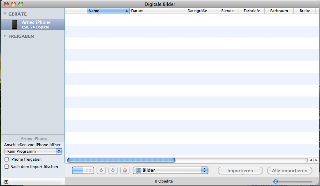## Tivoli Storage Manager: Servername not found

The infamous Tivoli Storage Manager client (a.k.a. dsmc) uses two configuration files: dsm.sys for the system wide configuration, specifying which directories to backup and so on, and the dsm.opt for the backup/restore client. The problem is, if you specify the backup SERVERNAME in both files, this will lead to problems, if they are not the same. So, e.g. I misconfigured the dsm.opt via the dsmj wizard, and had “SERVERNAME myhost” in the dsm.opt, while the dsm.sys said “SERVERNAME something.at.rwth-aachen.de”. The first entry obviously was complete bull, and confused the dsmc, so that it answered “ANS1217E Server name not found in System Options File”. Aligning both entries to the correct, real server name solves this problem.

## iPhone / iPhoto syncing woes

I just tried to download a couple of images from my iPhone, using iPhoto ’08. No luck. Suddenly the iPhone was not listed anymore in iPhoto! It turned out that something on the iPhone was broken / corrupt. When I looked at the device using the Image Capture program, I got this:As you can see the devices section lists 24 images on the phone, but the photo list is empty. Deleting the pictures on the device restored the functionality. If you need the photos badly, you can forward them to your email or MobileMe account, and then delete them.
Sometimes it is so much better to just have a plain filesystem with photos, instead of some advanced database, that might break…

## Bug in ff-find-other-file of Emacs 22?

I am working on a C++ project which is organized such that source files (*.cc) are in some subdir called, let’s say, project/dir1/, and header files (*.h) in a directory called project/include/. Now Emacs has a function called ff-find-other-file, which is able to switch between header and implementation of C/C++ files. If you take a look at the source of this function, you will find another function called ff-get-file-name, which tries the following: first, it looks for already visited buffers, if they contain the appropriate file. Then it will search a certain set of directories, if they contain the file. Last, it will ask to find or create the file in a user specified directory. However, in my case, although both files are already being visited by buffers, the first test (finding the file in already loaded buffers) fails. Actually the offending piece of code looks like this:

`   (if (bufferp (get-file-buffer filename))       (setq found (buffer-file-name (get-file-buffer filename))))`

The problem is that this always fails, and found will always be nil. The filename constructed is not being expanded, as get-file-buffer demands. However, expanding it is useless, since the exact expansion (absolute path) of the searched file is not exactly known! However, you can rework these lines to just look for a buffer whose name matches the file you are looking for:

`   (let ((b (find-if (lambda(x) (string= (buffer-name x) filename)) (buffer-list))))     (if b         (setq found (buffer-file-name b))))`

Note that this will only do a simple string matching. It might happen that for a certain file foo.cc you have to buffers visiting some foo.h. The function will only find the first one. But in my opinion, there is not simple solution to find the semantically correct header file, anyway. So this is still better than the broken solution in Emacs.

## Recursive Makefile for LaTeX

I wanted to make a Makefile for a seminar report. This report contains all the sub-reports of the students. So the Makefile needs to recurse into subdirectories to compile all the contained LaTeX files there. So, given a file reports.tex which will include some PDFs in subdirectories 00/ to 10/, we get a Makefile that looks like this:

`###################################################### A small LaTeX Makefile#####################################################PREFIX=reportsCHAPTERS=00 01 02 03 04 05 06 07 08 09 10all: subdirs \$(PREFIX).pdf#####################################################.PHONY: subdirs simplesubdirs:        @for i in \$(CHAPTERS); do               (cd \$\$i; \$(MAKE)); done#####################################################\$(PREFIX).aux:  \$(PREFIX).tex        pdflatex \$(PREFIX).tex\$(PREFIX).pdf: \$(PREFIX).aux        pdflatex \$(PREFIX).tex#####################################################simple:        pdflatex \$(PREFIX).tex#####################################################clean:        @for i in \$(CHAPTERS); do               (cd \$\$i; \$(MAKE) clean); done        rm -f \$(PREFIX).aux \$(PREFIX).bbl \$(PREFIX).blg          \$(PREFIX).idx \$(PREFIX).log \$(PREFIX).out \$(PREFIX).tcp          \$(PREFIX).toc \$(PREFIX).tps \$(PREFIX).prf \$(PREFIX).lbl        rm -f \$(PDFFILES) *.aux`

## Angry Birds

I have to make some advertisement for a funny little iPhone game: Angry Birds. The idea: green pigs stole some eggs, now the birds are angry! Use those Kamikaze birds as ballistic missiles to destroy the pigs! Nice physics engine. Also available on the N900, and costs only 79 Eurocents. Here’s a shot:## How to duplicate Matlab functionality

Since I am a total Matlab-n00b, I rather write a convoluted Python script instead of using the appropriate Matlab function. And here it is. A small script to compute the standard deviation of two vectors. I guess this would have been one Matlab command.

`import sys                                                                                                                                                                                       import scipy.io                                                                                                                                                                                  import math                                                                                                                                                                                                                                                                                                                                                                                      def computeStdDev(file, vec1, vec2):                                                                                                                                                                data = scipy.io.loadmat(file, struct_as_record=True)                                                                                                                                            vector1 = data[vec1]                                                                                                                                                                            vector2 = data[vec2]                                                                                                                                                                            if len(vector1) != len(vector2):                                                                                                                                                                    print "Error: Vectors do not have matching lengths."                                                                                                                                            return                                                                                                                                                                                      diff = vector1                                                                                                                                                                                  N = len(diff)                                                                                                                                                                                                                                                                                                                                                                                   for i in range(N):                                                                                                                                                                                  diff[i] = vector1[i] - vector2[i]                                                                                                                                                                                                                                                                                                                                                           mu = 0                                                                                                                                                                                          for val in diff:                                                                                                                                                                                    mu = mu + val                                                                                                                                                                               mu = mu / float(N)                                                                                                                                                                                                                                                                                                                                                                              sigma = 0                                                                                                                                                                                       for i in range(N):                                                                                                                                                                                  sigma = sigma + (diff[i] - mu)**2                                                                                                                                                           sigma = math.sqrt(sigma / float(N))                                                                                                                                                                                                                                                                                                                                                             print "mu: %f" % mu                                                                                                                                                                             print "sigma: %f" % sigma                                                                                                                                                                                                                                                                                                                                                                    if len(sys.argv) != 4:                                                                                                                                                                              print "Usage: %s file vector1 vector2" % sys.argv                                                                                                                                            sys.exit(0)                                                                                                                                                                                                                                                                                                                                                                                  computeStdDev(sys.argv, sys.argv, sys.argv)                                                                                                                                             `

## Matlab and Python

How to read Matlab files with Python and SciPy can be read here. Short:

`import scipy.iodata = scipy.io.loadmat('test.mat')`

This will create a dictionary called data containing all the variables of the Matlab file.